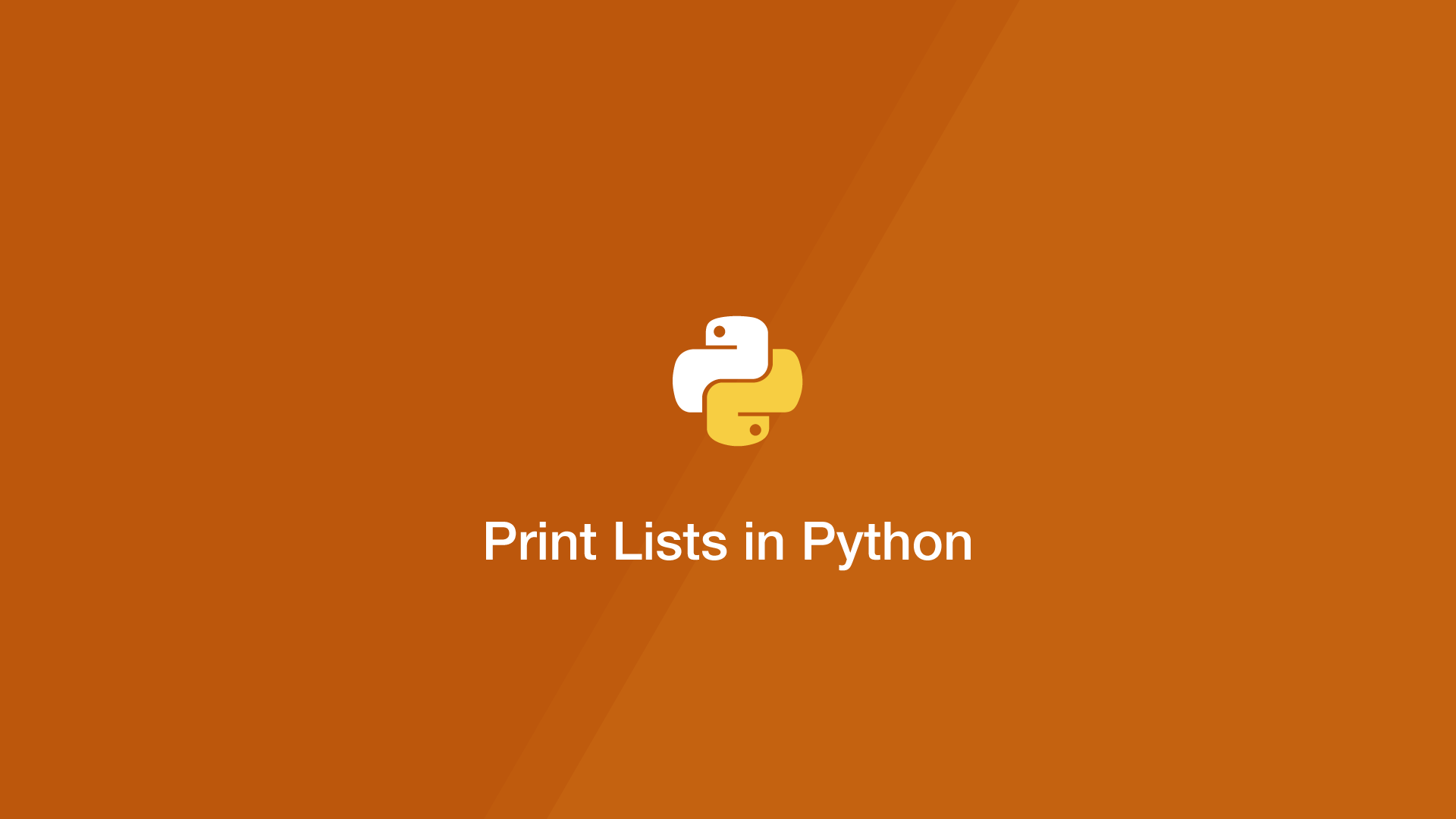# Print Lists in PythonThis tutorial will show the various ways of printing lists in Python.

The Python `map()` function loops through an iterable and executes a function on each element. To print a list as a string using `map()`, set the first argument as `str` and the second as the list. `map()` returns a map object so it will need to be converted to a string using the `.join()` method before printing.

``````items = ['a','b','c']

print(''.join(map(str, items)))
``````
``````abc
``````

A for loop iterates over every element in a list so we can use it to print a list like this:

``````items = ['a','b','c']

for i in items:
print(i)
``````
``````a
b
c
``````

## Use the * Operator to Print Lists in Python

As well as for multiplication, the `*` (asterisk) operator is used to output each element in a list separated by a space character.

``````items = ['a','b','c']

print(*items)
``````
``````a b c
``````

The Python `join()` method merges list elements into one string using a predefined separator. Here is an example of printing a list using join() with each element on a new line.

``````items = ['a','b','c']

print('\n'.join(items))
``````
``````a
b
c
``````

#### Related Tutorials### How to Create and Use Lists (Arrays) in Python

September 01, 2020### How to Calculate Mean, Median, Mode and Range in Python

October 07, 2020### How to use Sets in Python

September 15, 2020### How to Use Python List Comprehension

October 04, 2020### How to Use For Loops in Python

September 02, 2020### How to Round Numbers Up and Down in Python

September 28, 2020### The Python zip() Method

November 04, 2020